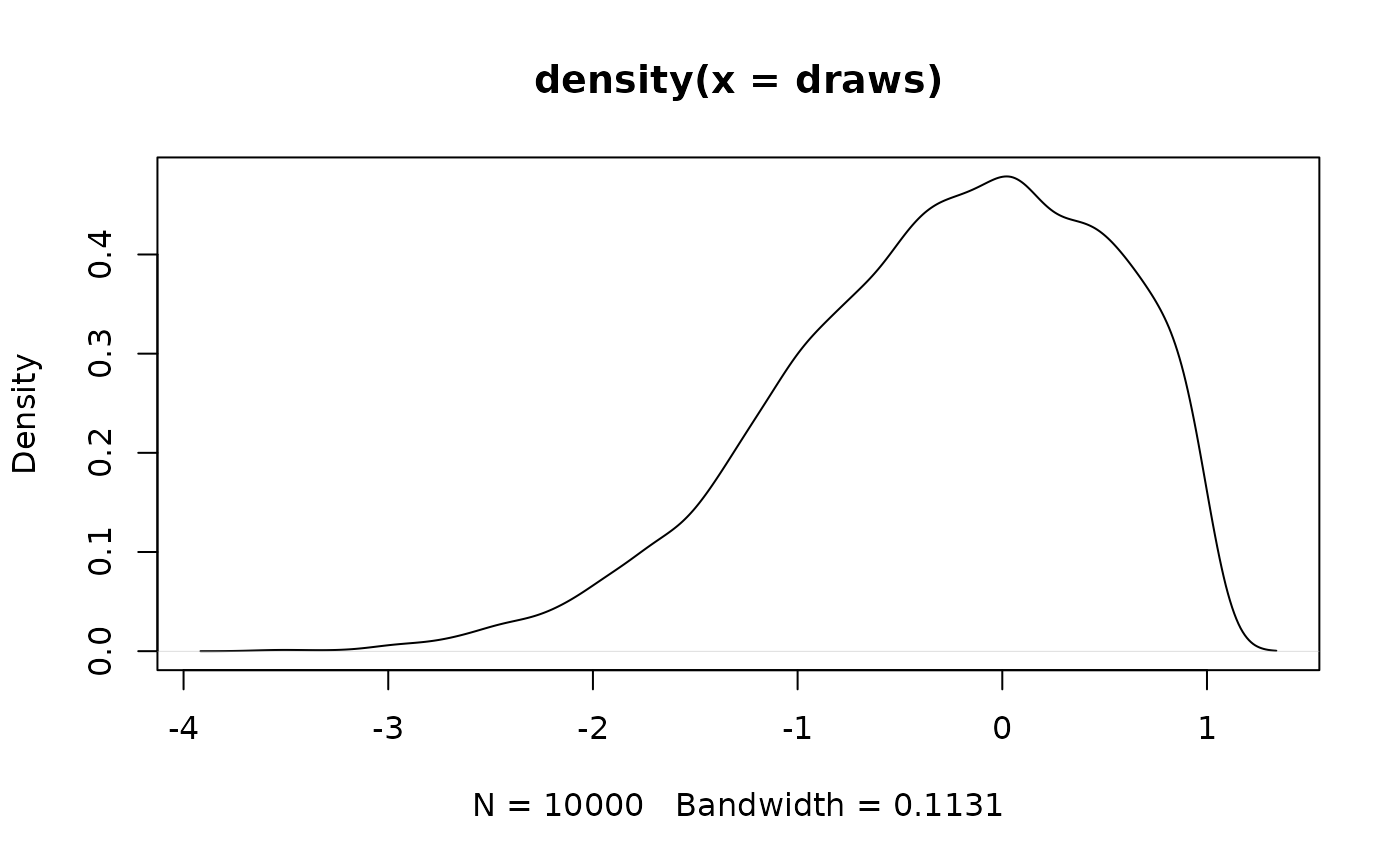This function draws from a one-sided truncated univariate normal distribution.

## Usage

rtnorm(mu, sig, trunpt, above)

## Arguments

mu

The mean.

sig

The standard deviation.

trunpt

The truncation point.

above

A boolean, if TRUE truncate from above, otherwise from below.

A numeric value.

## Examples

### samples from a standard normal truncated at 1 from above
R <- 1e4
draws <- replicate(R, rtnorm(0,1,1,TRUE))
plot(density(draws))